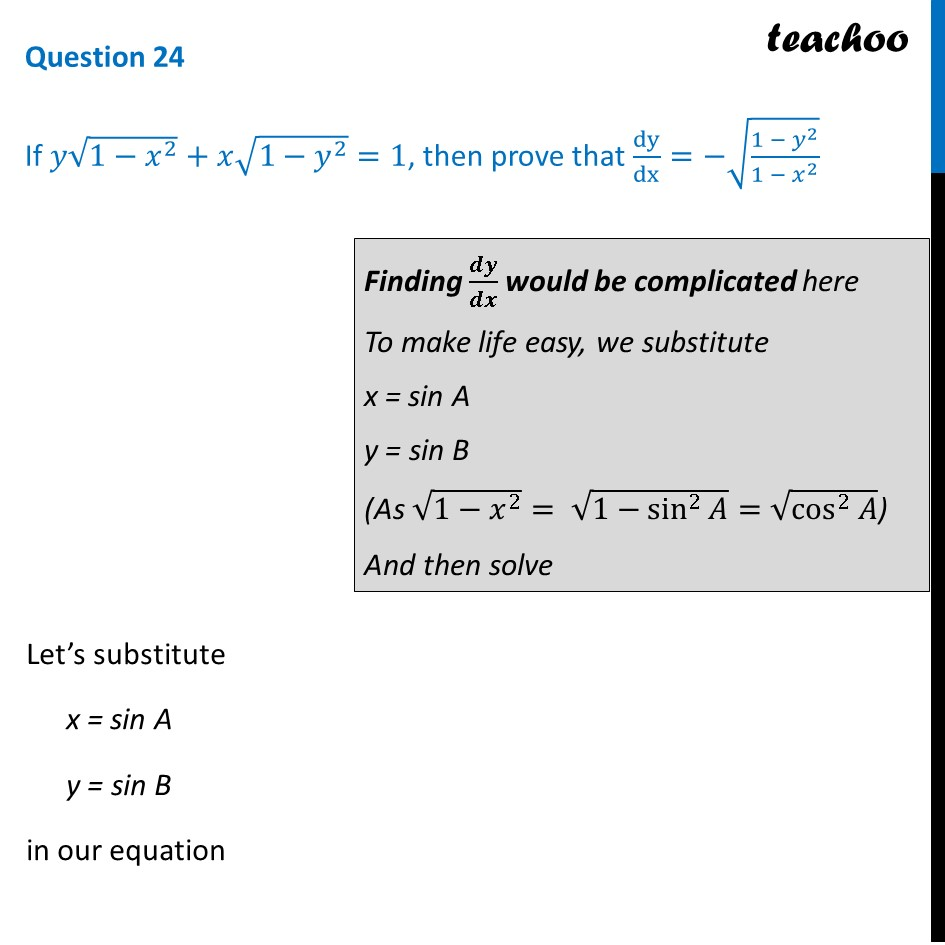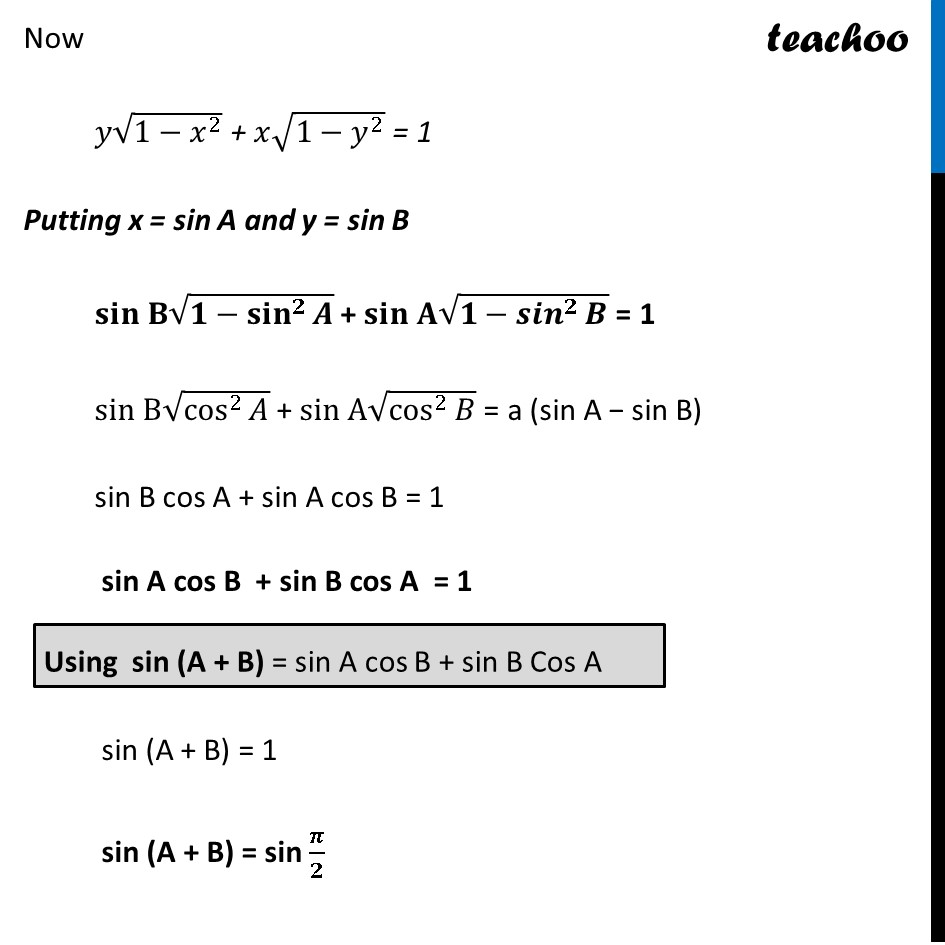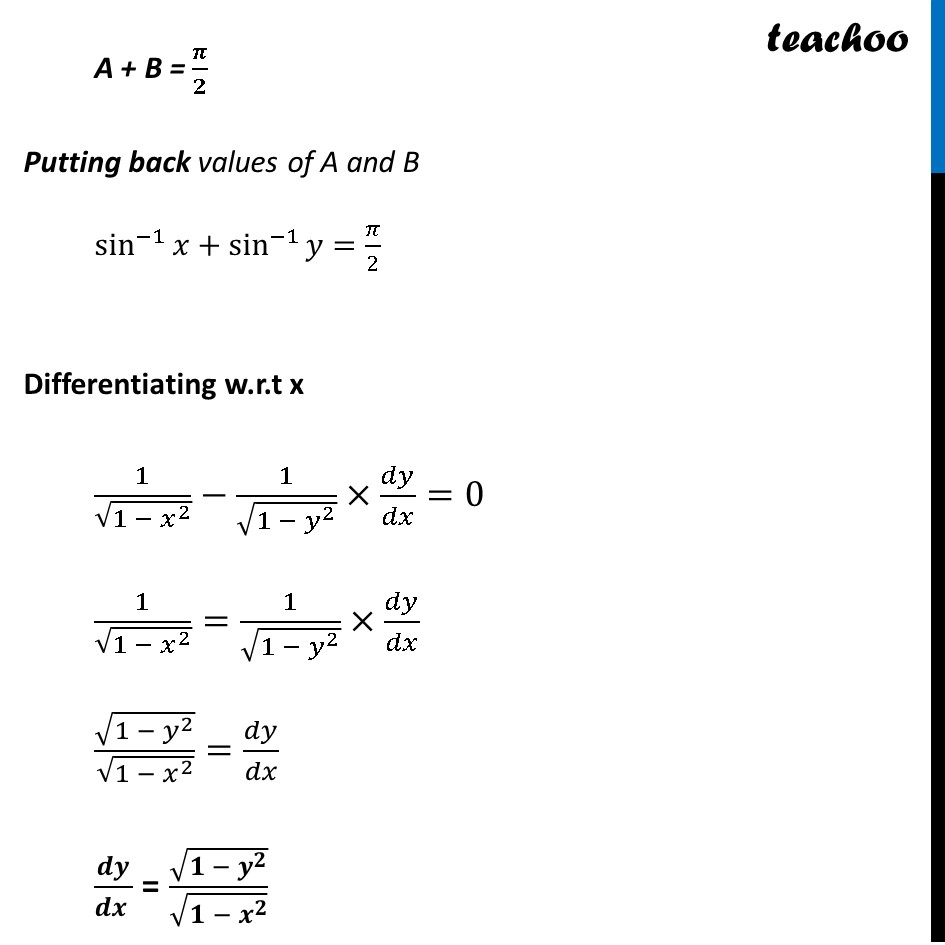CBSE Class 12 Sample Paper for 2023 Boards

Class 12
Solutions of Sample Papers and Past Year Papers - for Class 12 Boards

##googletag.cmd.push(function() { googletag.display('div-gpt-ad-1669298377854-0'); });(adsbygoogle = window.adsbygoogle || []).push({});Learn in your speed, with individual attention - Teachoo Maths 1-on-1 Class

### Transcript

Question 24 If 𝑦√(1−𝑥^2 )+𝑥√(1−𝑦^2 )=1, then prove that dy/dx=−√((1 − 𝑦^2)/(1 − 𝑥^2 )) Finding 𝒅𝒚/𝒅𝒙 would be complicated here To make life easy, we substitute x = sin A y = sin B (As √(1−𝑥^2 )= √(1−sin^2⁡𝐴 )=√(cos^2⁡𝐴 )) And then solve Let’s substitute x = sin A y = sin B in our equation Now 𝑦√(1−𝑥^2 ) + 𝑥√(1−𝑦^2 ) = 1 Putting x = sin A and y = sin B 𝐬𝐢𝐧 𝐁√(𝟏−〖𝐬𝐢𝐧〗^𝟐⁡𝑨 ) + 𝐬𝐢𝐧 𝐀√(𝟏−〖𝒔𝒊𝒏〗^𝟐⁡𝑩 ) = 1 sin B√(cos^2⁡𝐴 ) + sin A√(cos^2⁡𝐵 ) = a (sin A − sin B) sin B cos A + sin A cos B = 1 sin A cos B + sin B cos A = 1 sin (A + B) = 1 sin (A + B) = sin 𝝅/𝟐 A + B = 𝝅/𝟐 Putting back values of A and B sin^(−1)⁡𝑥+sin^(−1)⁡𝑦=𝜋/2 Differentiating w.r.t x 1/√(1 − 𝑥^2 )−1/√(1 − 𝑦^2 )×𝑑𝑦/𝑑𝑥=0 1/√(1 − 𝑥^2 )=1/√(1 − 𝑦^2 )×𝑑𝑦/𝑑𝑥 √(1 − 𝑦^2 )/√(1 − 𝑥^2 )=𝑑𝑦/𝑑𝑥 𝒅𝒚/(𝒅𝒙 ) = √(𝟏 − 𝒚^𝟐 )/√(𝟏 − 𝒙^𝟐 )